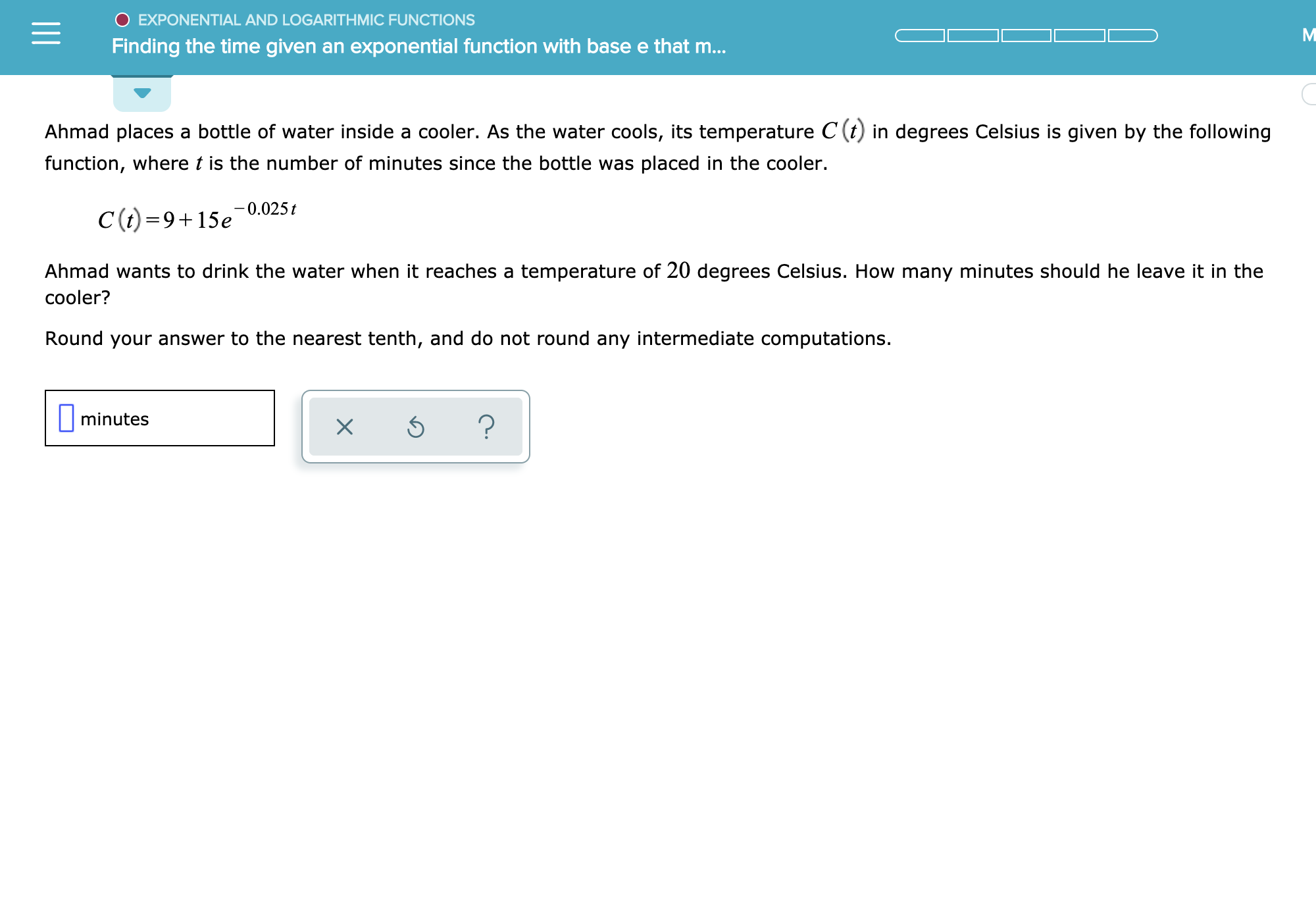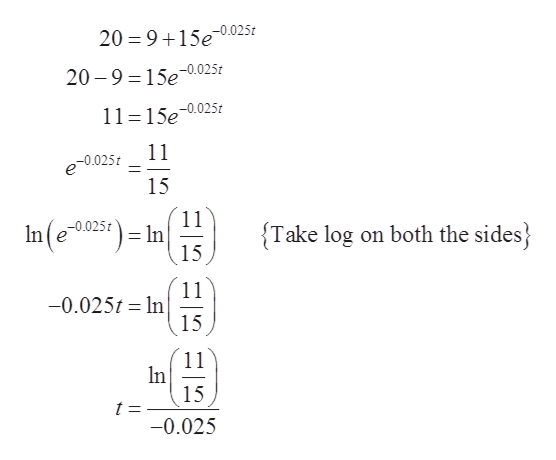# O EXPONENTIAL AND LOGARITHMIC FUNCTIONSFinding the time given an expoonential function with base e that m...Ahmad places a bottle of water inside a cooler. As the water cools, its temperature C (t) in degrees Celsius is given by the followingfunction, where t is the number of minutes since the bottle was placed in the cooler.C(t)=9+15e-0.025tAhmad wants to drink the water when it reaches a temperature of 20 degrees Celsius. How many minutes should he leave it in thecooler?Round your answer to the nearest tenth, and do not round any intermediate computations.minutes?X

Question
3 views

See attachmenthelp_outlineImage TranscriptioncloseO EXPONENTIAL AND LOGARITHMIC FUNCTIONS Finding the time given an expoonential function with base e that m... Ahmad places a bottle of water inside a cooler. As the water cools, its temperature C (t) in degrees Celsius is given by the following function, where t is the number of minutes since the bottle was placed in the cooler. C(t)=9+15e-0.025t Ahmad wants to drink the water when it reaches a temperature of 20 degrees Celsius. How many minutes should he leave it in the cooler? Round your answer to the nearest tenth, and do not round any intermediate computations. minutes ? X fullscreen
check_circle

Step 1

To determine the time t when the temperature of bottle inside the cooler is 20 degree Celsius.

Step 2

Given:

The temperature of the bottle in degree Celsius, t minutes after it is being place inside the cooler is given by the formula:

Step 3

Consider that the required temperature of the bottle is 20 degree Celsius.

Subs...help_outlineImage Transcriptionclose20 = 9 15e-0.025 20 9 150.025? 11 =15e-0.0252 11 e0.025 15 11 -0.025t- ln In (e025c) {Take log on both the sides 15 11 -0.025t n 15 11 n 15 t : -0.025 fullscreen

### Want to see the full answer?

See Solution

#### Want to see this answer and more?

Solutions are written by subject experts who are available 24/7. Questions are typically answered within 1 hour.*

See Solution
*Response times may vary by subject and question.
Tagged in
MathCalculus

### Functions